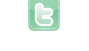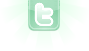Report as inappropriate.

# Top-down Survival Game

Controls:

Player 1: use WASD to move. "SPACEBAR" = attack, "V" = special attack, "SHIFT" = use item, "E" = pause.

Player 2: use the arrow keys to move. "APOSTROPHE" = attack, "L" = special attack, "SEMICOLON" = use item, "[" = pause.

Have fun surviving!

8257 views / 855 in the last 7 days

9 votes | 0 in the last 7 days

This scenario is a member of: Top Down Shooters

Your browser does not support the canvas tag.
In my games that are 8-directional, I usually import the provided SmoothMover class and move Math.sqrt((speed*speed)/2) in two directions. For a speed of two, for example, that would be the square root of two in two different directions to move two pixels diagonally, as (sqrt 2)^2 + (sqrt 2)^2 = 2^2, (Pythagorean theorem, the two bases (x,y) must be sqrt 2 to make the hypotenuse 2, the hypotenuse being the the diagonal distance moved). Sqrt 2 is a decimal number, so one must use SmoothMover or a similar system to track movement. Speed of 3 would be sqrt 4.5 in two directions (sqrt 4.5)^2 +(sqrt 4.5)^2 = 3^2 Speed of 4 would be sqrt 8 in two directions (sqrt 8)^2 +(sqrt 8)^2 = 4^2 And so on... For games that require 360 degree movement, I would use trigonometry. Sorry if my explanation is not too clear
Yeah, I am aware of this issue, but I never really thought it was major enough to remake the entire movement code to fix. I'm not sure if I'll ever get around to fixing it, to be honest. Sorry :(
Yes, in order to move diagonally the same distance as one pixel distance horizontally or vertically, you must multiply the distance by one half the square root of two, or approximately 0.7071; because that value squared is two-fourths, or one-half, and two halves is a whole. I do not know why you would complicate it by moving speed inside the square root function and squaring it when you can just leave it outside. That is, instead of 'Math.sqrt((speed*speed)/2)', you can just use 'speed*Math.sqrt(1/2)' or 'speed*Math.sqrt(2)/2'.
Hang on, it seems to me like you guys are complicating things. Could I not just use 'speed*Math.sqrt(2)' for diagonal movement?
@K_wow, see my last post. Another way of writing it could be 'speed/Math.sqrt(2)' (dividing, not multiplying).
Yes, I just put it as Math.sqrt((speed*speed)/2) to show a thought process. Speed*speed is c^2 in the Pythagorean Theorem, half of that is a^2 or b^2, and the square root of that is a or b, (only if the right triangle is isosceles of course). Any of the ways @danpost listed look neater and are more simplified; again, I only wrote it as I did to show a thought process. Again, sorry if I am making things more confusing.
Ah, yes. Of course 2 times the square root of two is not the same as the square root of eight.
@K_wow, they are equal: 2*sqrt(2) = sqrt ( (2^2) *2) = sqrt (4*2) = sqrt(8)
Oh, yeah. I mean 2 times the square root of two is not the same as the square root of four, but that's irrelevant. Even so, fixing diagonal movement is still pretty low on my priorities list right now.
A new version of this scenario was uploaded on Thu Sep 04 02:14:42 UTC 2014 Added settings menu. current settings are 'attack effects' and 'friendly fire'. Game over screen now shows the player's record this session. The scenario pause button now opens or closes the pause menu. Fixed bug where zombies are affected by the blue player's knockback in multiplayer. Fixed bug where the player could start a long assault rifle special attack by picking up the assault rifle while spinning with the sword. I'm taking a short break from this game to work on another simple greenfoot game that creates its images with code.